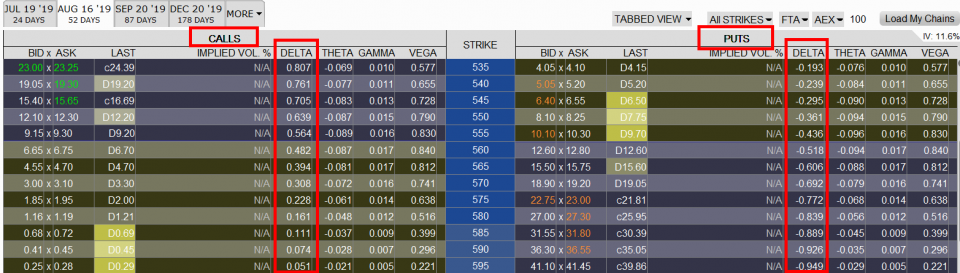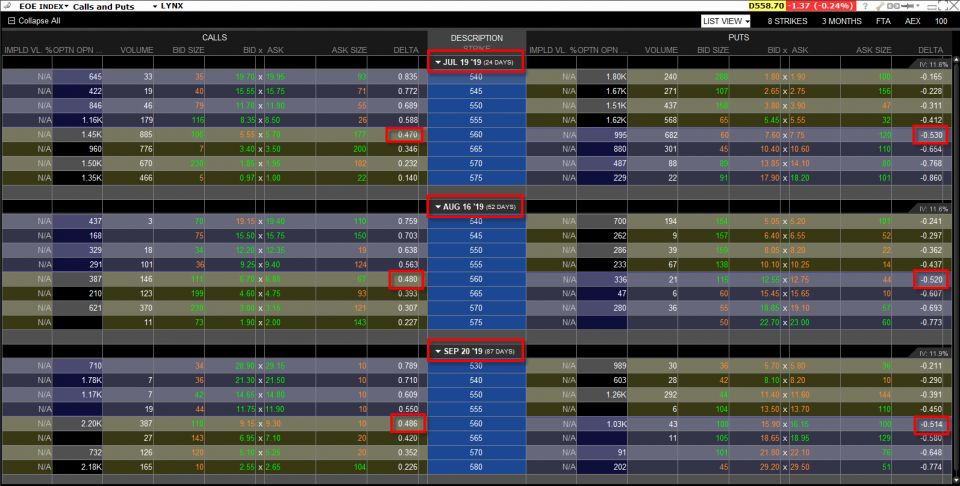Delta is one of the key Greek ratios used by option traders.

We will discuss the delta coefficient for options in detail below. After reading the article, you will better understand the meaning of the delta, learn about its features and influence the price of the option.

## What is delta?

Delta determines how the theoretical value of the option will change if the value of the underlying asset changes by one unit. In other words, it is a measure of the sensitivity of the option to the movement of the price of the underlying asset.

Put options have a negative delta coefficient, and call options have a positive delta coefficient. A positive delta means that the option price goes up as the underlying asset goes up and the option price goes down as the underlying asset goes down.

A negative delta means that the option price decreases as the value of the underlying asset increases, and the option price increases as the value of the underlying asset decreases. In the screenshot below, you can see the available options for the AEX (EOE) index. It’s easy to see that call options have a positive delta and put options have a negative delta.Options available for AEX (EOE) and Delta index in TWS platform. Source: Trader Workstation (May 13, 2022)

The in-the-money option always has a delta of around 0.5. The delta for the put option ranges from 0 to -1, while the delta for the call option ranges from 0 to 1.

The screenshot below shows the option on AEX (EOE) index. The delta of a call option with a strike price of 560 for each expiry date is approximately 0.48, and the delta of a put option with an strike price of 560 for each expiry date is approximately -0.52.Call options and put options on the AEX (EOE) and delta index in the TWS platform. Source: Trader Workstation (May 13, 2022)

## Delta coefficient for the portfolio

For each open position, you can calculate its delta. If you know the delta of your option position and multiply it by the number of option contracts, you will get the total delta for all open options in your portfolio. For example, if you have 2 call options on ABC stocks with a delta of 0.3 in your portfolio, the total delta position of these options in your portfolio is 0.6 (= 0.3 x 2). Note that delta is not a constant number but changes with time.

### Free investment e-book

Get a free e-book on trading options. Explore option types, trading strategies and much more!

## Delta coefficient and volatility

Here are some facts about the delta in relation to volatility:

• The delta for the put option is from 0 to -1
• The delta for the call option is from 0 to 1
• The sum of the deltas for call and put options with the same strike price and expiry date is always 1
• The delta for put in-the-money is around -0.5 regardless of the expiration date
• The delta for the in-the-money call option is around 0.5 regardless of the expiry date
• The increase in volatility causes the delta for the ITM (In the money) call option to drop to 0.5
• The increase in volatility causes the delta for the OTM (Out of the money) call option to increase to 0.5
• The decrease in volatility causes the delta for the ITM call option to increase above 0.5
• The decrease in volatility causes the delta for the OTM call option to drop below 0.5

## Neutral delta strategy

If there are several combinations of options in a portfolio, it is not always easy to get a clear picture of the stability of the entire portfolio. The “neutral delta” strategy aims to ensure that the value of your portfolio does not change as a result of changes in the price of the underlying asset.

In practice, delta neutrality is always difficult to achieve. The deltas of your positions change every day, so keeping them at a neutral level requires active and regular interventions.

### Delta-neutral wallet

An option portfolio has a neutral delta when its positive and negative delta positions are balanced. For such a portfolio, the sum of the deltas is zero. This means that the change in the price of the underlying instrument does not affect the valuation of the portfolio.

You can create a neutral delta option portfolio by taking long or short stock positions. However, this is not the only possibility. Examples of options strategies with a neutral delta without taking a position in stocks include, for example, iron condor and strangle.

Suppose you choose an action option strategy. Each share has a delta of 1. Suppose you have 100 shares of one company and your portfolio delta is +100 (100 shares * 1 delta). In such a situation, we are looking for an option position that will offset the positive delta of the portfolio. We need a transaction with a delta of -100. Most options contracts contain 100 shares. This means that for a portfolio the contract delta will be 100 * options delta. So the delta of an ITM put contract with a delta of -0.5 will be -50 (100 * -0.5). This means that to fully balance the equity portfolio, you will need 2 put contracts that will give a total of a delta of -100 (2 contracts * 100 shares * -0.5 deltas).

Due to the fact that contracts with different strike prices have a different delta, we can operate them in such a way as to achieve the expected result. For example, having 70 shares, we can balance them by buying 1 put contract with a delta -0.7. However, it should be remembered that the portfolio delta is neutral only when the option delta is the same as at the time of the transaction. As the price of the underlying instrument changes, the delta of the purchased contract will change, which will also change the portfolio delta.

When taking a position or creating option portfolios with a neutral delta, we do the same, looking for a position from which we get the opposite delta value. When buying a call option with a delta of 0.4, we will offset the position by buying a put option with a delta -0.4

What if you are short? We do the same, but taking into account that the short delta is the opposite (negative for call, positive for put). If you are executing your option strategy on a stock index, you can also obtain a neutral delta by taking a long or short position on futures.

### What are the advantages of a delta neutral strategy?

• It can be used for hedging purposes and for securing investments. As a result of this strategy, the portfolio is less susceptible to changes in the prices of the underlying products.
• In a neutral delta strategy, other elements that determine the price of an option can also be isolated. Examples are time value and implied volatility. On this basis, you can then create further option strategies, which, for example, react to the time value (theta).

### How to get a delta neutral wallet?

The positive delta of the portfolio to a neutral level will be reduced, for example, by:

• Purchase of a put option
• Sale of call options
• Opening short positions in stocks. It could also be a stock index futures instead of a stock.

On the other hand, the negative delta of the portfolio to a neutral level will be raised by:

• Purchase of a call option
• Sale of put options
• Opening long positions in equities. It could also be a stock index futures instead of a stock.

## Trading options via LYNX

Whether you are a day trader or a long-term trader, you can take advantage of the excellent offer of LYNX as we enable you to trade a wide variety of financial instruments such as stocks, ETFs, futures, options, CFDs and forex at favorable commissions. In addition, we regularly publish training webinars. At LYNX, you can trade in real time through professional trading platforms.

In addition, as part of the Investment Portal, we regularly inform you about current investment opportunities on the market and news from the investment world. In case of any doubts, you can use our customer support for free.

Sources used in the article:

BLEKEMOLEN, J. Delta: de optiegriek om te begrijpen. LYNX [online]. Posted on 13.05.2022 [cit. 27. 7. 2022]. The article is available here.

Trade on world stock exchanges

With an investment account through LYNX, investors have direct access to national and international stock exchanges in Europe, the USA and Asia. Trade 150 markets, 33 countries and 25 currencies. Find out more on the website: List of Exchanges and Products

Mkt Cap

Vol

Daily Maximum

Daily minimum

Displaying the greyhound